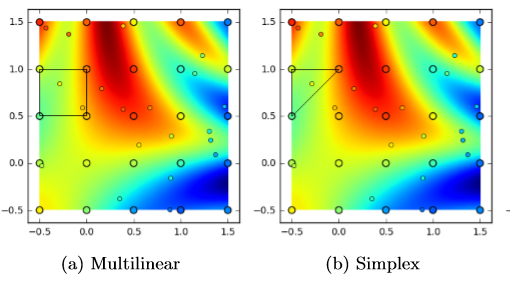dummy-link

32

5

10

11

# GridInterpolations

This package performs multivariate interpolation on a rectilinear grid. At the moment, it provides implementations of multilinear and simplex interpolation. As of benchmarks in December 2016, multilinear interpolation performs fastest and with the most accuracy.

The following image visualizes grid-based interpolation in two dimensions, with shape of interpolater for (−0.3,0.8) inscribed. The small dots reﬂect the interpolation's estimate for sin(x)+2cos(y)+sin(5xy), which is the underlying reward function approximated by the large dot lattice.For a description of multilinear and simplex interpolation see: Scott Davies, Multidimensional Triangulation and Interpolation for Reinforcement Learning, Advances in Neural Information Processing Systems, Cambridge, MA: MIT Press, 1997. pdf

There are some related packages, such as Grid.jl and Interpolations.jl.

## Installation

Start Julia and run the following command:

``````Pkg.add("GridInterpolations")
``````

## Usage

To use the GridInterpolations module, begin your code with

``````using GridInterpolations
``````

## Interpolation

Create two-dimensional interpolation grids, a data array, and a point of interest:

``````grid = RectangleGrid([0., 0.5, 1.],[0., 0.5, 1.])   # rectangular grid
sGrid = SimplexGrid([0., 0.5, 1.],[0., 0.5, 1.])    # simplex grid
gridData = [8., 1., 6., 3., 5., 7., 4., 9., 2.]     # vector of value data at each cut
x = [0.25, 0.75]                                    # point at which to perform interpolation
``````

Perform interpolation on the rectangular grid:

``````julia> interpolate(grid,gridData,x)
5.25
``````

Or interpolate on the simplex grid:

``````julia> interpolate(sGrid,gridData,x)
6.0
``````

Compute interpolants for the grids:

``````julia> sGrid = SimplexGrid([0., 0.5, 1.],[0., 0.5, 1.])
[[0.0,0.5,1.0],[0.0,0.5,1.0]]

julia> interpolants(sGrid, x)
([4,5,8],[0.5,0.0,0.5])
``````

Convert an index to a Grid coordinate:

``````julia> ind2x(grid, 3)
2-element Array{Float64,1}:
1.0
0.0
``````

Number of vertices in the grid:

``````julia> length(grid)
9
``````

Number of dimensions:

``````julia> dimensions(grid)
2
``````

Multi-dimensional indexing using Cartesian coordinates:

``````julia> [grid[c] for c in CartesianIndices((3,3))]
3×3 Array{Array{Float64,1},2}:
[0.0, 0.0]  [0.0, 0.5]  [0.0, 1.0]
[0.5, 0.0]  [0.5, 0.5]  [0.5, 1.0]
[1.0, 0.0]  [1.0, 0.5]  [1.0, 1.0]
``````

or multi-dimensional indices

``````julia> grid[2,2]
2-element Array{Float64,1}:
0.5
0.5
``````

Sequential iteration over grid points:

``````julia> for x in grid
# do stuff
end
``````

## Credits

Contributors to this package include Maxim Egorov, Eric Mueller, and Mykel Kochenderfer.

02/12/2015

7 days ago

71 commits